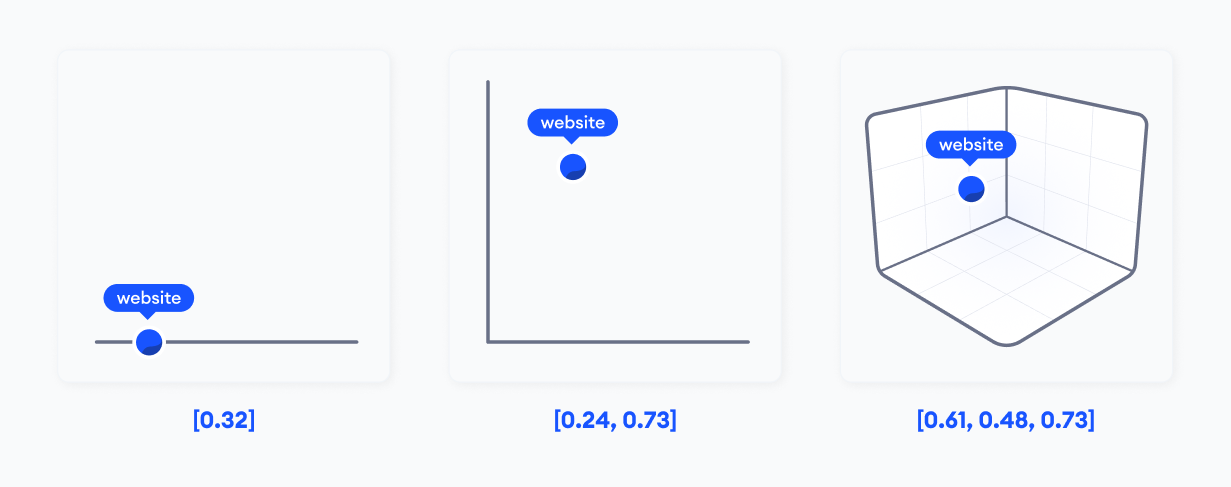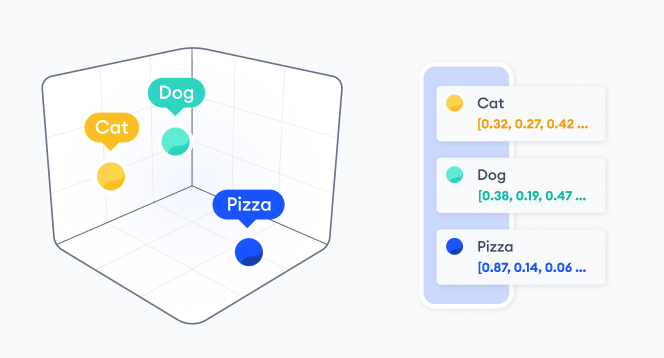# What are vectors

An introduction to vectors

### What are vectors?

Computers are unable to analyse unstructured data such as text, image, audio or geospatial data out of the box. To perform any sort of analysis, such as creating image based recommendation systems or text topic analysis, we need to convert them into a numerical format that a computer can analyse. By using machine learning, we can convert the unstructured data into a numerical formats and feed it into specialised vector algorithms for a variety of use cases.

This conversion from unstructured data to numerical data is called the encoding process. Each item, that could be a single word, an image, or an audio file, is encoded into a list of numbers: we call this list a vector. The encoding process can be done by different kinds of algorithms, notably if it is done through a deep learning algorithm it is often called a deep learning vector embedding. Once encoded into a vector, the longer it is in length, the more information is represented about the data it encoded.For example, let's imagine I want to convert the word 'website' to a vector. Shown in the graphics above, is the conversion of the word 'website' into vectors. We only visualise up to a length of 3 for the vector because human's can typically only visualize up to three dimensions (vector length of 3), but to properly encode a word that encapsulate as much of the word's semantic meaning, we may need vector length or dimension in the hundreds or thousands.
Although we cannot visualize it, this is an example of how a 6 dimension length vector of the word 'website' could look like:

``````website = [0.324, 0.241, 0.934, 0.424, 0.141, 0.242]
``````

Once we have these representation of data in the form of vectors, computer algorithms can compare the numerical values between multiple vectors to determine similarity between multiple unstructured data points. Similar to how your fingerprint or DNA is similar to people that are most similar to you: your parents or your siblings. Vector is the fingerprint of data, helping you find similarities within the data.

### What is a vector space?

When we encode thousands of words using the same machine learning algorithm, we project these words into the same vector space. This allow us to place words with similar meanings more closely.

In the example below, we have encoded 3 words: 'dog', 'cat', and 'pizza'. The word 'dog' and 'cat' are used in the same context very often and are semantically more similar, as opposed to 'cat' and 'pizza'. This was learnt by the machine learning model and when we produce a vector from it, we can see below that the words 'cat' and 'dog' are closer to each other and far way from 'pizza'.### 📘Vectors can capture semantic meaning and enable better similarity comparisons of data

By capturing all the different semantics and features of a data in a high dimensional vectors, we can use it to essentially perform better similarity analysis between data of any kind as long as it can be vectorized. By solving similarity we enable a wide array of use cases such as search, recommendations, predictions, etc.

In example above, the vectors were produce from BERT a NLP model from Google which produces a vector length of 768. For interpretation purposes, we have used a method of dimensionality reduction that compresses the vector length into 3 allowing the vector to be visualized.